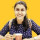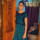1. Home >
2. Apps >
3. Groups >

# Transition and Diffusion capacitance in P-N junction diode

Question asked by Prajakta Kelapure in #Electronics on Jul 29, 2014Prajakta Kelapure · Jul 29, 2014
Rank D1 - MASTER

Transition and Diffusion capacitance

Transition capacitances:

1. When P-N junction is reverse biased the depletion region act as an insulator or as a dielectric medium and the p-type an N-type region have low resistance and act as the plates.

2. Thus this P-N junction can be considered as a parallel plate capacitor.

3. This junction capacitance is called as space charge capacitance or transition capacitance and is denoted as CT .

4. Since reverse bias causes the majority charge carriers to move away from the junction , so the thickness of the depletion region denoted as W increases with the increase in reverse bias voltage.

5. This incremental capacitance CT may be defined as

CT = dQ/dV,

Where dQ is the increase in charge and dV is the change or increase in voltage.

6. The depletion region increases with the increase in reverse bias potential the resulting transition capacitance decreases.

7. The formula for transition capacitance is given as CT = Aε/W, where A is the cross sectional area of the region, and W is the width.

Diffusion capacitance:

1. When the junction is forward biased, a capacitance comes into play , that is known as diffusion capacitance denoted as CD. It is much greater than the transition capacitance.

2. During forward biased the potential barrier is reduced. The charge carriers moves away from the junction and recombine.

3. The density of the charge carriers is high near the junction and reduces or decays as the distance increases.

4. Thus in this case charge is stored on both side of the junction and varies with the applied potential. So as per definition change in charge with respect to applied voltage results in capacitance which here is called as diffusion capacitance.

5. The formula for diffusion capacitance is CD = τID / ηVT , where τ is the mean life time of the charge carrier, ID is the diode current and VT is the applied forward voltage, and η is generation recombination factor.

6. The diffusion capacitance is directly proportional to the diode current.

7. In forward biased CD >> CT . And thus CT can be neglected.

Posted in: #ElectronicsDefyTheInstitution · Sep 4, 2015
Rank E2 - BEGINNER
Thank you, that was awesome!Ankita Katdare · Sep 8, 2015
Rank A1 - PRO
@Prajakta Kelapure It's great to know that the students are finding this so useful and informative. You should start writing tutorials again. 👍Akash Sharma · Oct 18, 2016
Rank E1 - BEGINNER
This is good.very useful .Jafer hameed · Sep 21, 2017
Rank E2 - BEGINNER
Great step forward
Like to know how can I post questionsJino John · Jul 17, 2018
Rank E1 - BEGINNER

It's too good .l learned a lot from thisPrasanth Prince · Nov 23, 2018
Rank E1 - BEGINNER

In diffusion capacitance 2nd point there is a mistake move away fromPrasanth Prince · Nov 23, 2018
Rank E1 - BEGINNER

It is towards the junctionKavya Burramolla · Feb 1, 2020
Rank C1 - EXPERT

Thanks @Prajakta Kelapure for the valuable information regarding diffusion and transition capacitance.

Addding a point :- Generally diffusion capacitance is smaller than the transition capacitance reason being that diffusion capacitance is caused by forward bias of the junction in the PN diode and transition capacitance is caused by the reverse bias of the junction in the PN junction diode.

Transition capacitance is basically the change of charge stored in the depletion region with respect to change in voltage. And Diffusion capacitance is the capacitance caused due to movement of charge carriers between from anode to cathode in forward biased mode.Ankita Katdare · Feb 20, 2020
Rank A1 - PRO

Can someone explain what is meant by space charge capacitance or junction capacitance of diode? Please provide diagram and explanatory image of equations in your answer.

## You must log-in or sign-up to reply to this post.

Click to Log-In or Sign-Up